# Pandas – Multi-index and groupbys

• Last Updated : 07 Mar, 2022

In this article, we will discuss Multi-index for Pandas Dataframe and Groupby operations .

Multi-index allows you to select more than one row and column in your index. It is a multi-level or hierarchical object for pandas object. Now there are various methods of multi-index that are used such as MultiIndex.from_arrays, MultiIndex.from_tuples, MultiIndex.from_product, MultiIndex.from_frame, etc which helps us to create multiple indexes from arrays, tuples, dataframes, etc.

Syntax: pandas.MultiIndex(levels=None, codes=None, sortorder=None, names=None, dtype=None, copy=False, name=None, verify_integrity=True)

• levels: It is a sequence of arrays which shows the unique labels for each level.
• codes: It is also a sequence of arrays where integers at each level helps us to designate the labels in that location.
• sortorder: optional int. It helps us to sort the levels lexicographically.
• dtype:data-type(size of the data which can be of 32 bits or 64 bits)
• copy: It is a boolean type parameter with default value as False. It helps us to copy the metadata.
• verify_integrity: It is a boolean type parameter with default value as True. It checks the integrity of the levels and codes i.t if they are valid.

Let us see some examples to understand the concept better.

Example 1:

In this example, we will be creating multi-index from arrays. Arrays are preferred over tuples because tuples are immutable whereas if we want to change a value of an element in an array, we can do that. So let us move to the code and its explanation:

After importing all the important libraries, we are creating an array of names along with arrays of marks and age respectively. Now with the help of MultiIndex.from_arrays, we are combining all the three arrays together such that elements from all the three arrays form multiple indexes together. After that, we are showing the above result.

## Python3

 `# importing pandas library from``# python``import` `pandas as pd` `# Creating an array of names``arrays ``=` `[``'Sohom'``,``'Suresh'``,``'kumkum'``,``'subrata'``]` `# Creating an array of ages``age``=` `[``10``, ``11``, ``12``, ``13``]` `# Creating an array of marks``marks``=``[``90``,``92``,``23``,``64``]` `# Using MultiIndex.from_arrays, we are``# combining the arrays together along``# with their names and creating multi-index``# with each element from the 3 arrays into``# different rows``pd.MultiIndex.from_arrays([arrays,age,marks], names``=``(``'names'``, ``'age'``,``'marks'``))`

Output: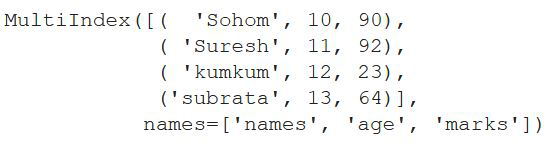Example 2:

In this example, we will be creating multi-index from dataframe using pandas. We will be creating manual data and then using pd.dataframe, we will create a dataframe with the set of data. Now using the Multi-index syntax we will create a multi-index with a dataframe.

In this example, we are doing the same thing as the previous example. The difference is that, in the previous example, we were creating multi-Index from a list of arrays whereas over here we created a dataframe using pd.dataframe and after that, we are creating multi-index from that dataframe using multi-index.from_frame() along with the names

## Python3

 `# importing pandas library from``# python``import` `pandas as pd` `# Creating data``Information ``=` `{``'name'``: [``"Saikat"``, ``"Shrestha"``, ``"Sandi"``, ``"Abinash"``],``               ` `               ``'Jobs'``: [``"Software Developer"``, ``"System Engineer"``,``                        ``"Footballer"``, ``"Singer"``],``               ` `               ``'Annual Salary(L.P.A)'``: [``12.4``, ``5.6``, ``9.3``, ``10``]}` `# Dataframing the whole data``df ``=` `pd.DataFrame(``dict``)` `# Showing the above data``print``(df)`

Output: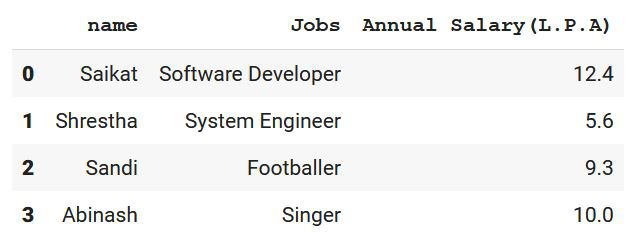Now using MultiIndex.from_frame , we are creating multiple indexes with this dataframe.

## Python3

 `# creating multiple indexes from``# the dataframe``pd.MultiIndex.from_frame(df)`

#### Output: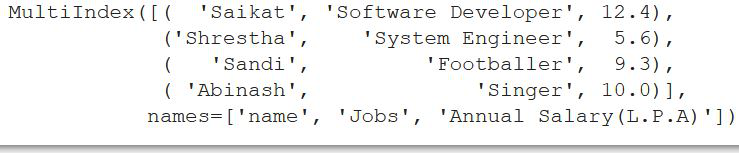Example 3:

In this example we will be learning about dataframe.set_index([col1,col2,..]), where we will be learning about multiple indexes. This is another concept of multi-index.

After importing the required library ie pandas we are creating data and then with the help of pandas.DataFrame we are converting it into a tabular format. After that using Dataframe.set_index we are setting some columns as the index columns(Multi-Index). Drop parameter is kept as false which will not drop the columns mentioned as index column and thereafter append parameter is used for appending passed columns to the already existing index columns.

## Python3

 `# importing the pandas library``import` `pandas as pd` `# making data for dataframing``data ``=` `{``    ``'series'``: [``'Peaky blinders'``, ``'Sherlock'``, ``'The crown'``,``               ``'Queens Gambit'``, ``'Friends'``],``    ` `    ``'Ratings'``: [``4.5``, ``5``, ``3.9``, ``4.2``, ``5``],``    ` `    ``'Date'``: [``2013``, ``2010``, ``2016``, ``2020``, ``1994``]``}` `# Dataframing the whole data created``df ``=` `pd.DataFrame(data)` `# setting first and the second name``# as index column``df.set_index([``"series"``, ``"Ratings"``], inplace``=``True``,``             ``append``=``True``, drop``=``False``)``# display the dataframe``print``(df)`

Output:Now, we are printing the index of dataframe in the form of multi-index.

## Python3

 `print``(df.index)`

Output: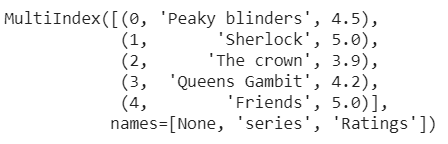## GroupBy

A groupby operation in Pandas helps us to split the object by applying a function and there-after combine the results. After grouping the columns according to our choice, we can perform various operations which can eventually help us in the analysis of the data.

Syntax: DataFrame.groupby(by=None, axis=0, level=None, as_index=True, sort=True, group_keys=True, squeeze=<object object>, observed=False, dropna=True)

• by: It helps us to group by a specific or multiple columns in the dataframe.
• axis: It has a default value of 0 where 0 stands for index and 1 stands for columns.
• level: Let us consider that the dataframe we are working with has hierarchical indexing. In that case level helps us to determine the level of the index we are working with.
• as_index: It is a boolean data-type with default value as true.It returns object with group labels as index.
• sort: It helps us to sort the key values. It is preferable to keep it as false for better performance.
• group_keys: It is also a boolean value with default value as true. It adds group keys to indexes to identify pieces
• dropna: It helps to drop the ‘NA‘ values in a dataset

Example 1:

In the example below, we will be exploring the concepts of groupby using data created by us. Let us move into the code implementation.

## Python3

 `# importing pandas library``import` `numpy as np` `# Creating pandas dataframe``df ``=` `pd.DataFrame(``    ``[``        ``(``"Corona Positive"``, ``65``, ``99``),``        ``(``"Corona Negative"``, ``52``, ``98.7``),``        ``(``"Corona Positive"``, ``43``, ``100.1``),``        ``(``"Corona Positive"``, ``26``, ``99.6``),``        ``(``"Corona Negative"``, ``30``, ``98.1``),``    ``],``    ` `    ``index``=``[``"Patient 1"``, ``"Patient 2"``, ``"Patient 3"``,``           ``"Patient 4"``, ``"Patient 5"``],``    ` `    ``columns``=``(``"Status"``, ``"Age(in Years)"``, ``"Temperature"``),``)` `# show dataframe``print``(df)`

Output: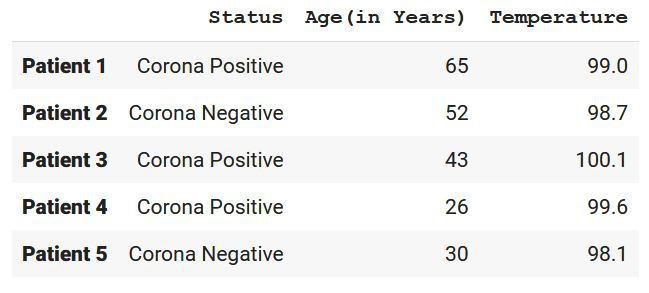Now let us group them according to some features:

## Python3

 `# Grouping with only status``grouped1 ``=` `df.groupby(``"Status"``)` `# Grouping with temperature and status``grouped3 ``=` `df.groupby([``"Temperature"``, ``"Status"``])`

As we can see, we have grouped them according to ‘Status‘ and ‘Temperature and Status‘. Let us perform some functions now:

## Python3

 `# Finding the mean of the``# patients reports according to``# the status``grouped1.mean()`

This will create the mean of the numerical values according to the ‘status‘.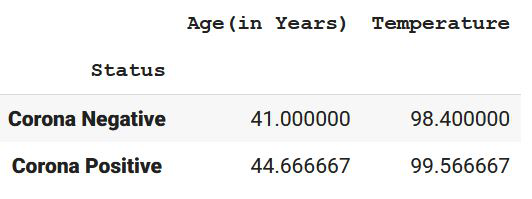## Python3

 `# Grouping temperature and status together``# results in giving us the index values of``# the particular patient``grouped3.groups`

Output:

{(98.1, ‘Corona Negative’): [‘Patient 5’], (98.7, ‘Corona Negative’): [‘Patient 2’],

(99.0, ‘Corona Positive’): [‘Patient 1’], (99.6, ‘Corona Positive’): [‘Patient 4’],

(100.1, ‘Corona Positive’): [‘Patient 3’]}

My Personal Notes arrow_drop_up